Previous

### The Firm

So far we have discussed production in the context of one person converting his time into some service such as lawn mowing. While that is a useful place to start, it ignores two important features of production--the use of more than one input in producing an output and the cooperation of more than one person in production. In this chapter, we will explore production in the more complicated case of the firm--a group of people combining inputs to produce an output.

Why do firms exist? Part of the reason is suggested by Figures 6-5 and 6-6 of Chapter 6--because two people coordinating their production can do better for themselves than if they act independently. In Chapter 6, the coordination occurred through trade. Individuals produced independently but, in deciding how much to produce of what, took into consideration the possibility of trading it for something else. In a firm, the cooperation is closer. Typically many individuals work together to produce a single item. The obvious reason is that they produce more that way. This is, in large part, a result of the principle of division of labor--if each of us specializes in a particular part of the productive process, he can be much better at it, hence more productive, than if each of us has to do everything. It is difficult to imagine one worker, however skillful and well equipped, producing an automobile in a year entirely by himself, yet an automobile factory produces several automobiles per worker per year.

In the production of automobiles, some of the division of labor occurs within the firm and some between firms; General Motors does not, for example, produce the steel from which its cars are made. One could imagine a society in which there was a high degree of division of labor, all of which occurred between firms, with each firm participating in only one part of the productive process and perhaps consisting of only one person. That possibility, and the difficulties it would create, are discussed in the optional section at the end of this chapter.

In discussing consumption, we reduced the individual to a set of preferences and his environment to a set of prices and a budget constraint. In discussing the firm, we follow a somewhat similar process--how similar will be clearer by the end of the chapter. We start with a production function, which describes the ways in which the firm can convert inputs (labor, raw materials, the use of machinery) into its output (the product it produces); we assume, for simplicity, that each firm produces only one product. The production function, plus the assumption that the firm is trying to maximize its profits, describe the firm; the prices the firm faces, for both its inputs and its output, describe its environment. The combination of the two tells us what the firm will do--how much it will produce and how.

### PART I - FROM PRODUCTION FUNCTION TO COST CURVES

We begin with a production function, which tells how a firm can transform its inputs into the goods it produces. You can think of a production function as an explicit function, Q(x1,x2,x3,. . .), where Q is the amount produced and x1, x2, . . . are the amounts of all the different inputs that can be used to produce it. Alternatively, you can think of a production function as a very large table listing all possible combinations of inputs and, for each combination, the resulting quantity of output. Table 9-1 is part of such a table for a firm manufacturing clay pots; the explicit production function is given at the bottom. Each row of Table 9-1 shows the number of pots that can be produced in a year with a particular collection of inputs--so much labor, so much use of capital, so much clay.

#### Table 9-1

 Input Bundle Labor (hours) Cost of Labor (\$10/hr Capital (\$-years) Cost of Capital (.05/yr) Clay (pounds) Cost of Clay (\$4/lb) Total Cost Output of Pots A 1.00 \$10.00 100 \$5.00 1.00 \$4.00 \$19.00 1 B 0.25 2.50 400 20.00 4.00 16.00 38.50 1 C 4.00 40.00 25 1.25 .25 1.00 42.25 1 D 2.00 20.00 200 10.00 2.00 8.00 38.00 2 E 4.00 40.00 100 5.00 1.00 4.00 49.00 2 F 1.00 10.00 100 5.00 16.00 64.00 79.00 2 G 1.00 10.00 1,600 80.00 1.00 4.00 94.00 2 H 3.00 30.00 300 15.00 3.00 12.00 57.00 3 I 9.00 90.00 100 5.00 1.00 4.00 99.00 3 J 4.00 40.00 100 5.00 5.06 20.24 65.24 3 K 4.00 40.00 225 11.25 2.25 9.00 60.25 3 L 1.00 10.00 8,100 405.00 1.00 4.00 419.00 3 M 4.00 40.00 400 20.00 4.00 16.00 76.00 4 N 9.00 90.00 178 8.89 1.78 7.12 106.01 4 O 0.946 9.46 94.6 4.73 1.18 4.72 18.92 1 P 1.89 18.90 189 9.45 2.36 9.44 37.84 2 Q 2.84 28.40 284 14.20 3.55 14.20 56.76 3 R 3.78 37.80 378 18.90 4.73 18.92 75.68 4
Output = Labor1/2 x (Capital/100)1/4 x Clay1/4

The table also shows the cost of the inputs; we assume that the firm is a small enough part of the market so that the prices at which it buys its inputs and sells its output are a given, not something affected by how much it buys or sells. The price of labor is \$10/hour, the price of clay is \$4/pound, and the price for the use of capital (strictly speaking, its rental) is .05/year. The meaning of the first two is obvious; the third, the price of capital, is an interest rate. If the interest rate is .05/year (or, more conventionally stated, "5% per annum"), then using \$100 worth of capital for a year costs you \$5--the interest you would pay if you borrowed the money to buy the \$100 worth of machinery that you must use for a year (\$100 x 1 year = 100 dollar-years of capital) in order to produce a pot using input bundle A. At the end of the year, you could either resell the machinery and pay back the loan or keep the machinery for another year and use another 100 dollar-years worth of capital to produce another pot--at a cost, for capital, of another five dollars interest on your loan. If you find it odd that your inputs consist of pounds of clay but dollar-years of capital, and that you have used capital as an input even if you give back the machine when you have finished with it, consider that exactly the same thing is true of the third input--labor. Your input is not workers but man-hours, and you return the worker (to himself) when you have finished employing him.

The firm must figure out how much to produce and how to produce it. A sensible first step, on the principle of dividing hard problems into manageable pieces, is to pick a level of output and figure out, given the production function and the prices of inputs, how to produce that quantity at the lowest possible cost. To do so, you start by considering all of the different combinations of inputs that would produce that quantity of output. On Table 9-1, for example, input bundles H, I, J, K, L, and Q can each be used to produce three pots. Next you calculate the cost of each bundle. This is just like calculating the cost of a consumption bundle; you multiply the quantity of each input by its price to find out how much you spend on that input, then add the figures for all the inputs to find the cost of the whole bundle, as shown on the table. Mathematically this gives us

C = P1x1 + P2x2 + P3x3 + . . .

C is the cost of that particular bundle. But there are usually many different combinations of inputs that will produce the same output. By using more labor, for example, you can minimize wastage and so reduce your consumption of raw material; whether that is worth doing depends on how expensive raw material is in comparison to labor. By using more machinery (ultimately capital--you will have to wait until Chapter 14 for a clearer explanation of what capital is), you may be able to economize on labor, raw material, or both. There are many kinds of raw material you can use (substituting among plastic, aluminum, and steel, for example, in making an automobile), and each of them comes in many different forms at different prices. Figuring out how to produce 73 television sets is an immensely complicated process with no single answer--there are many ways to do it. Typically, however, there is only one least expensive way, which is what the firm is looking for.

Comparing, on Table 9-1, the different bundles that can be used to produce one pot, you find that bundle O does it at the lowest cost; similarly bundle P is the least expensive way of producing 2 pots, Q of producing 3, and R of producing 4. Figure 9-1 shows the total cost curve (total cost as a function of quantity) implied by Table 9-1.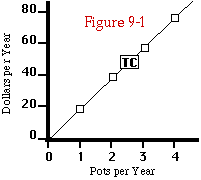Total cost for producing clay pots. The figure shows the cost of the least-cost bundle of inputs for producing each quantity of pots.

Since the table shows only a few of the possible ways of producing any particular number of pots, you cannot tell whether you have found the least costly input bundle or only the least costly of those shown. That is one of the problems with using a finite table to represent an infinite number of alternatives. In the optional section of this chapter, I show how one can use calculus to find the lowest cost bundles of inputs to produce various levels of output. The results of those calculations, for output levels of 1, 2, 3, and 4, are bundles O, P, Q, and R. They represent only a slight improvement over bundles A, D, H, and M, the lowest cost found without calculus. In this case, at least, one can come fairly close to achieving the perfectly rational decision by simple trial and error.

It may have occurred to you that the way in which we use Table 9-1 to find the least-cost way of producing pots is very similar to the way in which a similar table was used in Chapter 3 to find the most attractive consumption bundle. The logic of the two problems is almost exactly the same. In Chapter 3, we compared all the consumption bundles with the same cost to find out which produces the greatest utility; in this chapter, we compare all the input bundles producing the same output to see which has the lowest cost.

Having analyzed the production function, there are now two directions to go. We will first analyze the firm's behavior as a buyer, in order to deduce its demand curve for steel, labor, and the other inputs it uses in production. We will then analyze its behavior as a producer and seller, in order to deduce its supply curve for its output. As you will see, the two sides are connected, since the price for which the firm can sell its output is one of the things determining its demand for inputs.

The Input Market

Geometry I: Isoquant curves and Isocost lines. In Chapter 3, after recognizing that the table showed only a tiny sample of the possible consumption bundles, we went on to analyze the same problem geometrically, using budget lines and indifference curves. The same approach applied to production is shown on Figure 9-2; just as in the case of consumption, the fact that we are drawing our curves on two-dimensional paper means that we can only show two variables at a time. Here the two variables are inputs--labor and clay, used to produce pots. We may imagine either that there are only two or that we have already decided on the amount of the other inputs, such as capital, to be used.

In Chapter 3, the individual maximizes his utility subject to a budget constraint; here the firm minimizes its "budget"--its total expenditure--subject to a fixed level of production. These represent essentially the same process. The individual consumer tries to get as much of something (utility, happiness, "his objectives") as possible while spending a given amount of money; the firm tries to spend as little money as possible while getting a given amount of something (output). Figure 9-2 is the equivalent, for a firm, of the indifference curve diagrams of Chapter 3.

The contour Q1 is called an isoquant. It shows the different combinations of the two inputs that can produce a given quantity of output (73 pots). The blue lines are isocost lines; each shows all the input bundles that can be bought at a given cost. The isocost line is straight for the same reason the budget line is; we are assuming that the firm buying inputs, like the consumer buying consumption goods, can purchase as much as it wants at a constant price per unit.

In Chapter 3, we had a given budget line and were looking for the highest indifference curve that touched it. Here we have a given isoquant and are looking for the lowest isocost line that touches it. That is why the figures in Chapter 3 showed one budget line and several indifference curves, while here Figure 9-2 shows one isoquant and several isocost lines. In Chapter 3, the solution was to find the (indifference) curve that was tangent to the (budget) line; the optimal consumption bundle was at the point of tangency. Here the solution is to find the (isocost) line that is tangent to the (isoquant) curve; the optimal input bundle, the lowest cost bundle of inputs to produce that quantity of output, is at the point of tangency.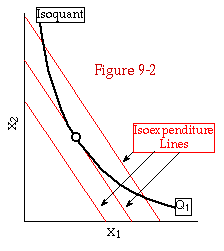Isoquant/isocost diagram for two inputs. Each isocost line shows the different bundles of inputs that have the same cost. The isoquant, Q1, shows the different bundles of inputs needed to produce a given quantity of output. The point of tangency is the optimal (i.e., lowest cost) input bundle for producing that quantity of output.

Suppose the firm has figured out the lowest cost way of producing a particular output: 73 television sets, a million cars, three pots, or whatever. It repeats the calculation for every other level of output it might consider producing: 74 television sets, 50 television sets, 900 television sets. At the end of the process, it has converted its production function into a total cost function--a function that tells it how much it will cost to produce any level of output.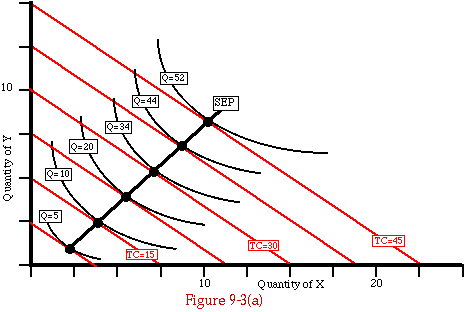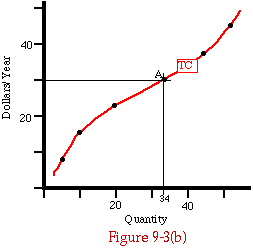Scale expansion path and total cost curve for the case of two inputs. The scale expansion path (SEP) in Figure 9-3a indicates the input bundles that would produce the various output quantities at the lowest cost. Figure 9-3b shows the resulting total cost curve.

Figures 9-3a and b show how this would work for the case of two inputs, X and Y, with prices Px=2, Py=3. I have labelled the isoquants and some of the isocost lines on 9-3a. The points of tangency show the input bundles that would produce the various output quantities at the lowest cost. Line SEP, which links those points, is the scale expansion path; it shows how the consumption of the inputs X and Y would increase as output expanded. Figure 9-3b shows the resulting total cost curve. Point A on Figure 9-3b, for instance, shows total cost of 30 for producing a quantity of 34. It corresponds to point a on Figure 9-3a, where the isoquant for producing 34 units is tangent to the isocost line for a cost of 30.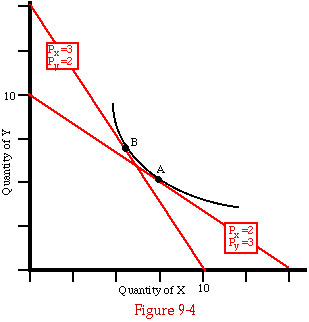The effect of a change in input prices. When the price of X rises and the price of Y falls, theoptimal input bundle for producing 34 widgets changes from A to B. The firm substitutes the input that has become cheaper for the input that has become more expensive.

Figure 9-4 shows how the inputs used to produce a given quantity of output (34 widgets) would change if the price of the inputs changed. Point A shows the input bundle that produces 34 widgets at lowest cost if Px is 2 and Py is 3; point B shows the lowest cost bundle if we reverse the prices. As one would expect, when the prices change, the firm shifts to using more of the good that has become cheaper (Y) and less of the good that has become more expensive (X). This factor substitution effect is precisely analogous to the substitution effect in consumption described in Chapter 3. There we were rolling a budget line along an indifference curve, here we are rolling an isocost line along an isoquant.

In Chapter 3, we were able to use figures similar to this (3-8a and 3-9a) to derive the individual's demand curve for a good. Can we, in the same way, derive the firm's demand curve for its input?

The answer is no. Figure 9-4 shows the inputs that the firm would use to produce a given quantity of output at different input prices. But as input prices change, so does the cost to the firm of producing its output, and hence the quantity that it chooses to produce. We would have to take account of that effect if we wanted to derive demand curves for inputs.

How would we do so? We have just seen how, for a given set of input prices, the firm calculates a total cost curve. In the next section we will see how, from the total cost curve and the market price, the firm decides how much to produce. In order to calculate the firm's demand curve for one input, say steel, we would simply repeat this analysis for a range of different steel prices, holding the prices of all other inputs fixed. For each price we would calculate the profit-maximizing quantity of output (say automobiles), and for that quantity we would calculate the quantity of steel in the least-cost bundle of inputs.

I Knew I Had an Equimarginal Principle Lying Around Here Somewhere. The same argument that led us to the equimarginal principle in consumption applies in production as well, if we replace marginal value with marginal product--after first defining the latter. The marginal product of an input is the rate at which output increases as the quantity of that input increases, all other inputs held constant. You may think of it as the increase in output resulting from one additional unit of that input. If adding one worker to a factory employing 1,000, while keeping all other inputs fixed, results in an additional 2 cars per year, then the marginal product of labor is 2 cars per man-year.

How can you produce two more cars with no more steel? The answer is that for small variations in inputs, one factor can substitute for another--in this case, labor for raw materials. One of the things the additional labor may do is improve quality control, so that fewer cars have to be scrapped; another is to make possible a more labor-intensive production process that produces cars with slightly less steel in them. Table 9-1 shows the same thing happening with the manufacture of pots. As you go from bundle A to bundle E, for example, the amount of clay and of capital used stay the same; the amount of labor quadruples, and the output rises from 1 pot to 2. Perhaps what is happening is that in A, many of the pots crack when they are fired; in E, the workers are spending four times as much time on each pot, and as a result they have doubled the percentage that survive firing. Going from A to E, labor input increases by 3 man-hours and output by 1 pot, so the marginal product of labor is 1/3 pot/man-hour.

If we consider large changes in inputs, this becomes less plausible--it is hard to see how one could produce either pots or cars with no raw materials at all, however much labor one used. This is an example of the law of diminishing returns, which plays the same role in production as does the law of declining marginal utility in consumption. If you hold all factors but one constant and increase that one, eventually its marginal product begins to decline. Each additional man-year of labor increases the number of cars produced by less and less. However much fertilizer you use, you cannot grow the world's supply of wheat in a flowerpot. In just the same way, as you hold all other consumption goods fixed and increase one, eventually the additional utility from each additional unit becomes less and less. I will not trade my life for any number of ice cream cones.

The equimarginal principle in consumption tells us that if you have chosen the optimal consumption bundle, the value to you of an additional dollar's worth of any good in the bundle--anything you consume--is the same. The equimarginal principle in production tells us that if the firm is minimizing its costs for a given quantity of output, the additional output produced by a dollar's worth of any input it uses is the same. Algebraically, if MPx is the marginal product of input x and similarly for input y, we have:

MPx/Px=MPy/Py.

The argument, which should seem familiar, goes as follows: If the firm is already producing its output at the lowest possible cost, there can be no way of reducing its cost any further while producing the same quantity of output. Suppose there are two inputs whose marginal product per dollar's worth is different--an additional dollar's worth of input A increases output by 4 units; an additional dollar's worth of input B increases output by 3 units. To reduce cost while producing the same amount of output, use \$0.75 more of input A and \$1 less of input B. Output goes up by (4 units/\$'s worth) x (3/4 \$'s worth) = 3 units, because of the increased input of A. It goes down by 3 units because of the decreased input of B. So the net effect is to produce the same output with \$0.25 less expenditure--which is impossible if the firm is already producing at minimum cost.

If you find it confusing to use a "dollar's worth" as a unit to measure the quantity of input, we can use physical units instead. Input A costs \$1/pound and its marginal product is 4 units per pound; input B costs \$2/pound and its marginal product is 6 units per pound. Use 3/4 of a pound more of A, 1/2 pound less of B; output remains unchanged and expenditure has fallen by \$0.25.

In this case, just as in the similar proof in Chapter 4, the argument only works precisely if the amount of the change is infinitely small, so that the marginal product of an input is the same whether we consider an increase or a decrease. The larger the size of the changes in inputs we consider, the less precise the argument becomes. But in order to prove that the firm is not producing at minimum cost, all we need show is that there is some change that lowers cost while maintaining output--even a very small change will do.

We have shown that if the marginal product of a dollar's worth of input is not the same for all inputs, or in other words if the marginal products of inputs are not proportional to their prices, it is possible to alter the bundle of inputs in such a way as to reduce cost while maintaining the same output. It follows that for the least-cost bundle--the bundle of inputs that a profit-maximizing firm will choose--the marginal products of inputs are proportional to their prices. That is the equimarginal principle in production.

If you look back at Figure 9-2, you will see that we could have derived the same result there. The slope of the isocost line is the ratio of the prices of the two inputs. The slope of the isoquant at any point is the ratio of the marginal products of the two inputs: the marginal rate of substitution in production. It shows the rate at which inputs substitute for each other in the production function (how much you must increase one input to balance the output loss due to a unit decrease in another) just as the marginal rate of substitution in consumption showed the rate at which consumption goods substitute for each other in the utility function. At the point of tangency, the two slopes are equal: Px/Py=MPx/MPy. If, in equilibrium, it requires a two-unit increase in input B to make up for a one unit decrease in input A, then input A must cost exactly twice as much per unit as input B.

Income comes from owning factors of production, such as your own labor, savings, land, or the like. The amount of income you get from the factors you own depends on how much you can sell or rent them for. The equimarginal principle in production, which tells us the relation between the prices of factors and their marginal product, will turn out to be of considerable interest when we discuss the distribution of income in Chapter 14.

Geometry II: Marginal Revenue Product and the Input Demand Curve. Just as in the case of consumption in Chapter 4, we can carry the argument one step further. The marginal product of an input, say steel, is measured in units of output: 1/2 automobile/ton of steel, say. The marginal revenue product (MRP) of the input is its marginal product (sometimes called its marginal physical product) multiplied by the additional revenue the firm gets for each additional unit produced--the price at which it sells its output. If an automobile sells for \$10,000 and an additional ton of steel increases output by half an automobile, then the marginal revenue product of steel is \$5000/ton.

Suppose steel costs only \$4000/ton. If the firm uses an additional two tons of steel while holding all other inputs constant, its production cost increases by \$8000, its output increases by one automobile, its revenue increases by \$10,000, and its profit increases by \$2000. As long as the cost of steel is lower than its marginal revenue product, profit can be increased by using more steel. So the firm continues to increase its use of steel until the marginal revenue product of steel equals its price: MRP=P.

The argument should be familiar--it is the same one used in Chapter 4 to show that P=MV. There is one essential difference. Consumption goods are bought with money but used to produce utility. In order to go from marginal utility to marginal value, we needed to know the rate at which the consumer could convert dollars into utiles, which depended on the tastes and opportunities of that particular consumer. Input goods are bought with money and used to produce output goods--which are then sold for money. We could not predict how many dollars worth of utility the consumer would get from a given quantity of apples, but we can predict how many dollar's worth of automobiles a firm will produce with a given quantity of steel. The rate at which the firm can convert automobiles into dollars is simply the price of automobiles.

This is one example of a more general difference between the analysis of individuals and of firms. While we assume that individuals have relatively simple objectives, we do not know just what those objectives are. The objective of the firm, on the other hand, is known--at least, it is known in economic theory, and the theory seems to do a reasonably good job of explaining the real world. The objective of the firm is to maximize its profit. From that assumption plus the firm's opportunities, as embodied in its production function, the prices of its inputs, and the price at which it can sell its output, we can calculate what the firm will do.

In Chapter 4, we used the relation P=MV to derive the individual's demand curve from his marginal value curve. It would seem that we could, in exactly the same way, use the relation P=MRP to derive the firm's demand curve for its inputs. We simply draw the MRPs curve, showing marginal revenue product of steel as a function of its quantity. At any price, the firm buys that quantity of steel for which Ps=MRPs. So the firm's demand curve for steel, Ds, equals the MRPs curve, as shown on figures 9-5a and b.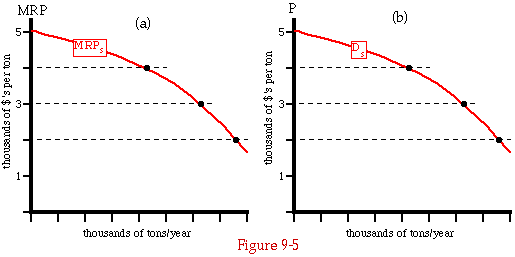The Marginal Revenue Product curve for steel and the demand curve for steel.

There is one problem with this. The marginal product of one input depends on the quantity of other inputs. In order to draw the curve MRPs on Figure 9-5a, I must first discover, for any quantity of steel, how much of each other input--rubber, labor, capital, etc. --the firm would choose to use.

To answer that question we again use the equimarginal principle. We know that, in equilibrium, the marginal physical product per dollar's worth of every input the firm uses must be the same. So for any given amount of steel, we use the production function to find the quantities of the other inputs at which the ratio of marginal product to price is the same for all inputs. At those quantities, we calculate the marginal revenue product of steel and put it on the graph.

Why did we not do the same thing in Chapter 4, when we were calculating the demand curves for consumption goods? In principle, we should have. Where two goods are closely related in consumption--bread and butter, for example, or gasoline and automobiles--the demand curve for one must take account of that relation. To calculate the marginal value of bread one must allow for the fact that as you increase your consumption of bread you also increase your consumption of butter--otherwise (assuming that you only like buttered bread) your marginal value for bread would drop off rapidly as you ran out of butter.

But in the case of consumption, such interdependencies are the exception, not the rule, so we could and did ignore them in Chapter 4--especially since, at that point, we were trying to describe a very simple economy. In the case of production, the interdependency of inputs is far more important--the marginal product of steel drops off very rapidly if you cannot hire additional laborers to make it into cars.

Warning. You should not interpret what we have done so far in this chapter as implying that an actual firm, say General Motors, has a list somewhere describing every possible way of producing every conceivable quantity of output and a room full of computers busy twenty-four hours a day figuring the least costly way of doing so. GM is profoundly uninterested in the cost of producing seven automobiles per year or 7 billion, and equally uninterested in the possibility of making them out of such inputs as bubble gum, lettuce, or the labor services of phrenologists.

Just as in the case of consumer behavior, our assumption is that people (and firms) tend to end up making the right decision, which in this case means producing goods at the lowest possible cost so as to maximize their profit. To figure out what that decision is, we imagine how it would be made by a firm with complete information and unlimited ability to process it. In practice, the decision is made by a much more limited process involving a large element of trial and error--but we expect that it will tend to produce the same result. If it does not, and some other practical method does, then some other firm will produce cars at lower cost than GM. Eventually GM will either imitate its competitor's method or go out of business.

The Output Market: Cost Curves

Figure 9-1 showed the total cost function for the pottery of Table 9-1. A total cost function is enormously simpler than a production function, since it has only one variable; you may, if you wish, think of it as the production function for producing automobiles (or anything else) using only one input--money. The single input is used to hire labor and machinery and to buy steel, glass, and other inputs, which are then used to produce automobiles. For most of the rest of this chapter, it is only the cost function and not the full production function that we will need in order to understand the firm's behavior.

Figure 9-6 shows total cost as a function of output for a hypothetical firm producing widgets. Fixed cost (FC) is the height of the total cost curve where it runs into the vertical axis--total cost as we approach zero output from the right. For the firm shown by TC2, total cost goes to zero as output goes to zero. For the firm whose total cost curve is TC1, the ability to produce anything at all involves a substantial cost (FC1). An example of such a fixed cost would be the cost of designing a new computer, which the firm must pay whether it is going to produce a million computers or only one.

One of the things not shown on the figure is the influence of time on costs. Cost of production really depends on rate of production (automobiles per year) as well as on amount of production (automobiles); the cost of producing 1,000,000 automobiles in ten years is very different from the cost of producing them in one year. The cost of producing different levels of output also depends on how much time the firm is given to adjust to changes. If GM has been producing 5,000,000 cars per year and suddenly decides to reduce output to 2,000,000, there will be many costs it cannot get out of--expensive factories standing empty, executives with long-term contracts who must be paid whether or not there is any work for them, and so on. If GM decides that over the next ten years its output will gradually fall to 2,000,000/year, it can gradually reduce its scale of operations to something more appropriate to the new rate of production.

Going in the other direction, if GM wishes to double its rate of output over a period of a few months, it will find it difficult and expensive; factories will be running all night, workers will have to be paid for overtime, and suppliers will have to be paid premium prices to get them to provide large quantities at short notice. If the same increase occurs gradually over a period of years, the cost is much less. In general, we would expect the total cost curve to rise more steeply with increasing quantity, and fall more gently with decreasing quantity, for short-term changes than for long-term changes. Curve TCSR on Figure 9-6a shows such a pattern, with A the point at which the firm is presently producing.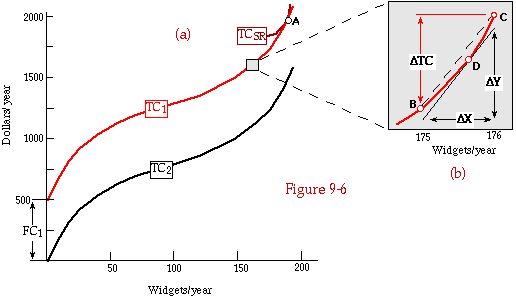Total cost curves with (TC1) and without (TC2) fixed cost. 6b is an expanded view of the section of 6a inside the square, showing the relation between the precise definition of marginal cost (slope of total cost) and the approximate definition (increase in total cost with a one-unit increase in quantity).

While the distinction between long-run and short-run cost curves is worth noting at this point, it need not be dealt with here. At this point, we are still considering a perfectly static and predictable world in which tomorrow is always just like today. In such a world, production decisions are made once and for all and never changed. The cost curves in this chapter describe costs for a firm that expects to produce the same quantity of output in the same way forever. In Chapters 12 and 13, we will finally drop that assumption. At that point, it will be necessary to return to the distinction between long-run and short-run cost curves; until then, we can ignore it.

Figure 9-7 shows the marginal cost curve corresponding to TC1 on Figure 9-6a. The relation between total cost and marginal cost is the same as the relation between total utility and marginal utility, total value and marginal value, or total product (quantity of output) and marginal product. Marginal cost is the rate at which total cost changes with output; it may be thought of, somewhat imprecisely, as the increase in total cost when output is increased by one unit. Just as marginal value is the slope of the total value curve, so marginal cost is the slope of the total cost curve.

Expressed in numbers, marginal cost at an output rate of 1,000 is the difference between the cost of producing 1,001 units and the cost of producing 1,000. Just as with marginal value, you should not try to associate marginal cost with a particular identifiable unit--a particular car, say. All the cars rolling off the assembly line are identical; the marginal cost of a car is the cost of making the total number of cars coming off that line larger by one.A marginal cost curve. MC is the marginal cost curve corresponding to TC1 in Figure 9-6.

Figure 9-6b is an expanded view of the part of Figure 9-6a inside the square; it shows the relation between the precise definition of MC (the slope of TC) and the approximate definition (increase of cost with a one-unit increase in quantity). The slope of TC1 at point D is [[Delta]] Y/[[Delta]] X. The increase in cost per unit increase in quantity, the slope of the dashed line BC, is [[Delta]] TC/[[Delta]] X. [[Delta]] X is one unit. The solid and the dashed line are almost exactly parallel, so their slopes are almost exactly equal.

So far, I have defined total cost (TC) and marginal cost (MC). There is a third kind of cost curve that we will find useful--average cost (AC). The average cost to produce any quantity of output is simply the total cost divided by the quantity; if it costs \$10,000 to produce 500 widgets, then the average cost is \$20/widget. Figure 9-8 combines curves from Figures 9-6a and 9-7 and adds AC, putting all three cost curves on one graph so as to make it easier to see the relations among them.

One thing you may notice about AC on Figure 9-8 is that it goes to infinity as quantity goes to zero. Why? As quantity goes to zero, total cost does not; the firm whose cost curves we are looking at has some fixed costs. Average cost is total cost divided by quantity; as quantity goes to zero, total cost approaches FC, so TC/q goes to FC/0--infinity. Figure 9-9 shows TC, MC, and AC for the firm represented by TC2 on Figure 9-6; there are no fixed costs, and AC does not go to infinity as quantity goes to zero.Total cost, marginal cost, and average cost for a firm. Because the firm has positive fixed cost, average cost goes to infinity as quantity goes to zero. Average and marginal cost intersect at point H, which is the minimum of average cost.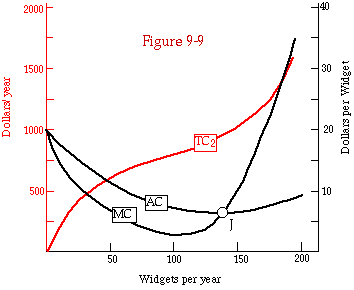Total cost, marginal cost, and average cost for a firm with no fixed cost.

There is also a useful relation between AC and MC that you may have noticed on Figures 9-8 and 9-9. Where marginal cost is above average cost, average cost is rising; where marginal cost is below average cost, average cost is falling. Where marginal cost is equal to average cost, at points H on Figure 9-8 and J on Figure 9-9, average cost is neither rising nor falling; it is horizontal.

Why? At an output of 150 widgets on Figure 9-8, total cost is \$1,500 and marginal cost is \$10/widget. Average cost is \$1,500/150 widgets = \$10/widget. If you increase output to 151, total cost increases by \$10--that is what MC = \$10 means. But if the average is \$10 and you increase quantity by 1 and cost by \$10, the average stays the same; you are averaging in one more unit whose cost is exactly the average of the previous units. If average cost does not change when you add another unit, then average cost is independent of quantity--the line is horizontal, as at points H and J.

Consider a different point on the graph, one at which output is 100 widgets, total cost is \$1,250, and marginal cost is \$3/widget. Average cost is \$1,250/100 widgets = \$12.50/widget. If you increase output to 101, total cost increases by \$3--that is what MC = \$3 means. But if the present average is \$12.50 and you increase quantity by 1 and cost by \$3, the average must fall. You are averaging in one more unit whose cost is less than the average of the previous units, so you are pulling the average down. The same thing would happen if you calculated the average height of a basketball team and then decided to average in the coach as well.

If MC is below AC, each additional unit of output pulls down the average. If an increase in quantity lowers average cost, then the AC curve is falling. So when marginal cost is below average cost, average cost is going down. Similarly, if marginal cost is higher than average cost, then increasing quantity means adding more expensive units to the average, which pulls the average up. So if marginal cost is above average cost, average cost is rising (getting higher as output gets higher).

Average cost is rising when it is below marginal cost, falling when it is above marginal cost, and level when it is equal to marginal cost. Now that you know the pattern, you should be able to see it easily enough on Figures 9-8 and 9-9. You should also be able to see that when average cost is at its minimum, it intersects marginal cost.

Why? Just before it reaches its minimum, average cost is falling; just after, it is rising. When it is falling, marginal cost must be below it; when it is rising, marginal cost must be above it. So marginal cost must cross average cost from below just at the minimum of average cost. A similar argument demonstrates that at a maximum of average cost, marginal cost crosses it from above. Running the same argument in the opposite direction, it is easy enough to show that these are the only two situations in which marginal cost can cross average cost; if the two curves cross, it must be at a minimum or maximum of average cost.

Students who try to memorize these relations frequently find them confusing; there are, after all, three different curves involved (TC, MC, AC) and two different kinds of characteristics (above/below, rising/falling). A better policy is to go over the argument until you can reproduce it for yourself, then do so when necessary. There are lots of relations that could exist among the curves, but only a few rather simple ones that do. While at this point they may seem to be the sort of thing that only a professor or textbook author could find of interest, they turn out to be surprisingly useful. In Chapter 16, the fact that marginal cost intersects average cost at the latter's minimum turns out to be a key element in the proof of what may be the most surprising, and important, result in all of economics. Stay tuned.

#### PART 2 - FROM COST CURVES TO SUPPLY CURVES

We have now derived the cost curves of the firm from its production function and the prices of inputs. The next stage is to use the cost curves to derive the firm's supply curve--the relation between the price at which it can sell its output and the amount it chooses to produce. The final step in the analysis is to combine the supply curves of many firms into a supply curve for the entire industry; doing this will turn out to involve some additional complications.

#### The Firm's Supply Curve

In Chapter 4, we derived the demand curve of the consumer from his marginal value curve; now we will use almost exactly the same argument to derive the supply curve of the firm from its marginal cost curve. Figure 9-10a shows the same curves as Figure 9-8; the only addition is the price P at which the firm can sell its output. We assume (as I mentioned earlier) that the firm, like individual producers in Chapter 5, is producing only a small fraction of the industry's total output, so that its decision of how much to produce has a negligible effect on P; from the firm's point of view, it can sell as much as it wishes at the market price and nothing at any higher price. For the same reason, we assume that the quantity of inputs the firm buys has no significant effect on the price it must pay for them. Each input has a market price; the firm can buy as much as it likes at that price and none at any lower price. These assumptions--that the firm cannot affect the price it can get for its output or the price it must pay for its input--are the central features of what economists call perfect competition. The effects of dropping those assumptions will be discussed in the next chapter.

The firm considers producing a quantity, q1, at which MC is lower than price. If it increased its output from q1 to q1 + 1, it would sell one more unit, increasing its revenue by P and its cost by MC. Since P is larger than MC, revenue would go up by more than cost, so profit, which is revenue minus cost, would increase. Obviously q1 is the wrong amount to produce. The same argument applies at q2. It continues to apply as long as marginal cost is less than price--MC < P. So the firm should expand its output up to the point at which MC = P. That is q3 on Figure 9-10a.

If a firm always produces that quantity for which MC = P, then its supply curve--the amount it produces as a function of price--is equal to its MC curve, just as a demand curve is equal to an MV curve for a consumer. This is almost correct, but not quite. Typically, MC first falls (as the increasing size of the firm produces advantages--more efficient production on a larger scale), then rises (the firm has taken full advantage of large-scale production; further increases in size mean more and more levels of administration between the president and the factory floor, leading to less efficient production). There may be prices at which, rather than producing a quantity for which MC = P, the firm prefers not to produce at all, thus saving the expense of producing units for which MC is higher than P. This occurs when, at the "optimal" quantity of output (MC = P), profit is still negative. One would get a similar effect with a demand curve if MV, instead of sloping steadily downward as shown on Figure 4-4, first rose and then fell. There would be prices at which, rather than consuming the quantity for which MV=P, the consumer would prefer to consume nothing in order not to have to pay for units whose marginal value was less than their price.

The firm's profit is the difference between what it takes in (total revenue--the quantity produced times the price for which it is sold) and what it spends (total cost). If there were no fixed cost, then total profit from producing quantity q3 on Figure 9-10a would be the colored area F minus the shaded area G. Starting at an output level of zero and expanding output up to qo, each additional unit costs more to produce than the price it sells for, contributing a (negative) profit of P - MC; adding all those little rectangles together gives us the area G. If the firm chose to produce a quantity qo, its profit would be minus G. As it continues expanding output beyond qo, the additional units sell for more than they cost to produce; again each unit increases profit by P - MC--but this time it is positive, since between qo and q3 marginal cost is less than P. The profit from expanding output from qo to q3 is the sum of all those little rectangles--the colored area F. So the total profit from producing a quantity q3 is F - G.

Seen this way, it becomes obvious why producing the quantity for which P = MC results in the maximum profit. If you produce less, you are giving up the opportunity to produce units that will sell for more than they cost to produce; if you produce more, expanding output to q4, the additional units cost more than they sell for, lowering profit by the area H. The argument should be familiar; it is essentially the same as the derivation of consumer surplus in Chapter 4.

So far, we have calculated what profit would be if there were no fixed cost. Fixed cost is the amount you have to pay in order to produce anything at all; it does not depend on how much you produce. Total cost is fixed cost plus variable cost: TC = FC + VC. Since fixed cost does not depend on how much you produce, it has no effect on the marginal cost curve, which shows the additional cost of producing one more unit. It does affect the average cost curve, since average cost is total (including fixed) cost divided by quantity. And since profit is total revenue minus total cost, fixed cost also comes out of profit. So if we include the effect of fixed cost, profit on Figure 9-10a is F - G - FC.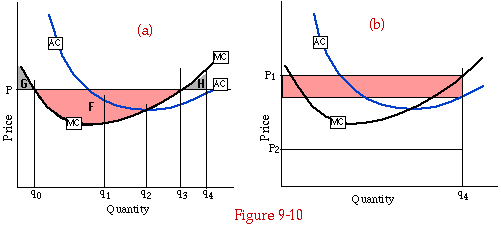The effect of quantity on profit. If the firm produces q3, where MC = P, profit is maximized. Expanding output to q4 decreases profit by H; contracting output to qo decreases profit by F. Figure 9-10b shows another way of calculating profit--as quantity x (price - average cost).

Earlier I showed that the firm, if it produces at all, maximizes its profit by producing that quantity for which MC = P. It may have occurred to you, looking at Figure 9-10a, that there are two quantities, (q0 and q3) for which MC = P. How does the firm decide which it should produce? The answer should be clear from the previous few paragraphs. If, in the region between the two points, the marginal cost curve is below the price line, then producing those units will increase profit--by area F on Figure 9-10a. So the firm is better off producing q3 instead of qo. If, in the region between the two points where marginal cost equals price, the marginal cost curve is above the price line, then the firm is losing money on those units and would be better off producing the lower quantity. As you should be able to see for yourself, this implies that the firm should produce a quantity at which the marginal cost curve crosses the price line from below--as it does at q3 on Figure 9-10a.

Figure 9-10b shows another way of calculating profit--one that can be done from the figure without knowing the size of fixed cost. Average cost is, by definition, total cost divided by quantity. So total cost is average cost times quantity. Total revenue is price times quantity. So profit--total revenue minus total cost--is simply quantity times the difference between price (P1) and average cost; it is shown as the shaded area on Figure 9-10b. That makes sense--price is what you get for each unit produced and average cost is what it costs you to produce it, so price minus average cost is your per-unit profit. Multiply that by quantity and you have total profit.

So profit is negative when price is below average cost; the firm would do better by shutting down entirely, eliminating its fixed cost by selling off all its facilities, and going out of business. If profit is negative for all quantities the firm could produce--if, in other words, the average cost curve is everywhere above the price line, as it would be if the price were P2 on Figure 9-10b--the firm's optimal decision is to go out of business and produce nothing--or better yet, never to come into existence in the first place. Whether or not that situation exists depends both on the firm's cost curves and on the market price.

We now know, for any price, how much the firm will produce. We have deduced the firm's supply curve. The firm produces nothing if the price is below the minimum of average cost. If price is above minimum average cost then there is some range of output quantity for which the firm can make positive profits; the firm maximizes its profit by producing the quantity for which marginal cost equals price. So the firm's supply curve is the rising portion of its marginal cost curve above its intersection with average cost. Figure 9-11a shows a series of different prices, P1, P2, P3, P4, and for each, the quantity the firm chooses to produce. Figure 9-11b shows the resulting supply curve.

On Figure 9-11b, and on similar figures in Chapter 5 and later in this chapter, the horizontal section of the firm's supply curve is shown as a dashed line. This is to indicate that the supply curve does not really exist in that region; if price equals minimum average cost, the firm will produce either nothing at all or the quantity for which average cost is minimum--making a profit of zero in either case. It will not produce any intermediate quantity, since that would result in negative profit.

The analysis we have just used to demonstrate the relation between the firm's marginal cost curve and its supply curve is the same used earlier to show that the individual's demand curve was equal to his marginal value curve. The only important difference is that we assumed marginal value always fell with quantity, while we expect marginal cost to first fall, then rise; the result is that the firm may have to produce over a range of output at which it is losing money on each additional unit (between zero and qo on Figure 9-10a, where marginal cost is greater than P) in order to reach a level of output where it is making money on additional units.

In this section, I have derived an important relationship linking the cost curves of the firm to its supply curve and to the amount of profit it makes. We will use these results repeatedly in this chapter and later in the book; you may want to go over the analysis again to be sure you understand it before continuing.

You may also find it useful to see how the analysis of the individual producer in Chapter 5 fits into this chapter as a special case--a one-person firm using a single input. The individual producer of Chapter 5 also had a supply curve that was equal to a marginal cost curve--the marginal cost to him of his own time. I explained the horizontal segment of a firm's supply curve by saying that below some price, the profit from producing is negative, so it is better not to produce. I explained the horizontal segment of the individual supply curve by the existence of a price for one good below which the producer is better off producing something else.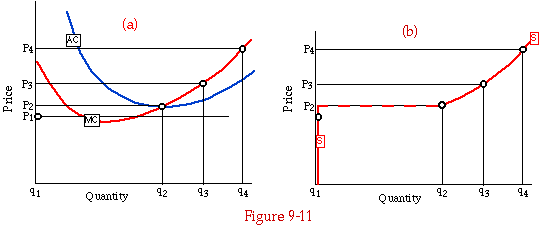Deducing a supply curve from a marginal cost curve. Figure 9-11a shows, for each price, the profit-maximizing quantity. Figure 9-11b shows the resulting supply curve, S.

The two explanations seem different, but they are not. One cost of using your time to dig ditches is that you are not cooking meals at the same time. How great is that cost? It is equal to what you could make by cooking meals. If the hourly return from digging is less than the hourly return from cooking, then digging produces a negative profit--when the opportunity cost of not cooking is taken into account. In Chapter 5, it was convenient to think of the "cost of working" as the "disvalue of labor"--sore muscles, boredom, and the like. But that is only one example of a more general sort of cost. The cost of mowing lawns is whatever you give up in order to do so, whether that is the pleasure of lying in bed reading science fiction books or the income from washing dishes.

Industry Supply Curve: Closed Entry

We now know how to derive the supply curve of a firm from its cost curves. The next step is to go from the supply curve of a firm to the supply curve of an industry made up of many firms. In doing so, we will encounter a number of complications. I will start with the simplest case and build up from there.

We begin with an industry made up of ten identical firms. We assume that the number of firms is fixed by law; it is illegal for anyone to start a new one. Figure 9-12 shows the supply curve for a single firm, Sf, the supply curve for the industry, Si, and the demand curve. Si is simply Sf multiplied horizontally by ten--the number of firms. If, at a price P, a single firm produces a quantity qf, then ten firms will produce 10 x qf. We are adding together, horizontally, ten identical supply curves, just as we added supply curves in Chapter 5. To find the market price, we simply look for the intersection of the supply curve and the demand curve, as in Chapter 7. It occurs at point E on Figure 9-12.

A number of points are worth noting here. The first is that although price is independent of output from the standpoint of the firm, the same is not true from the standpoint of the industry. The output of any single firm is too small to affect the price significantly, so each firm takes the price as given and adjusts quantity accordingly. But the output of the industry as a whole does affect price. If all the firms increase output, price falls; if all the firms decrease output, price rises. In Chapter 11, we will see what happens if the firms act together to restrict output and drive up price. In this chapter, we assume that the number of firms is sufficiently large so that each individual firm merely concerns itself with its own output and takes the behavior of the other firms as given.

If there are only ten firms, that assumption is a somewhat dubious one. I used ten firms in my example because for much larger numbers it becomes difficult to plot the firm supply curve and the industry supply curve on the same graph, as I did for Figure 9-12. You should really think of the analysis as applying to an industry with many more firms--hundreds or thousands of them. That is why, in drawing industry supply curves, I ignore the complications associated with small quantities of output--where there can be one firm producing, or two, but not one and a half.Deducing an industry supply curve from a firm supply curve in an industry with closed entry. The industry has ten identical firms. Its supply curve, Si, is the horizontal sum of ten firms' supply curves, Sf. The figure assumes that the quantity of inputs used by the industry has no effect on their price.

In deriving the firm's supply curve, we assumed that both the price at which it sold its output and the prices at which it bought its inputs were unaffected by the firm's decisions. While this is a reasonable assumption from the standpoint of one firm in the industry, it is less reasonable for an entire industry. If one farmer decides to double the amount of wheat he plants, he need not worry about the effect of that decision on the price of fertilizer or the wages of farm laborers; but if every farmer decides to double his planting of wheat, both fertilizer prices and farm wages are likely to rise.

It may seem inconsistent to say that no firm affects the price of its inputs but that the industry, which is made up of all the firms, does. It is not. From the standpoint of a single firm in an industry containing many firms, the effect of its demand on the price of inputs may well be negligible, so it can ignore that effect in deciding how much to produce. The same is not true for the industry as a whole. Each increase in the purchases of one firm causes a small increase in prices, which must be paid by all the other firms as well; this is called a pecuniary externality (an externality is a cost or benefit imposed by one firm or individual on another) and will be discussed in Chapter 18. The effect on one firm of the increased price of inputs caused by the increase in that firm's consumption may be negligible, while the effect on all of the firms of the increased price of inputs caused by the increased consumption of all of the firms is not.

Figure 9-12 takes no account of any such effect. It was drawn on the (unstated) assumption that the cost of the industry's inputs was unaffected by the amount of them that the industry bought--or, in other words, that the supply curve for the inputs is horizontal. This assumption is reasonable for some inputs to some industries--increased production of watches is not likely to have much effect on the price of steel, although steel is used in making watches--but not for all.

Figures 9-13a and 9-13b show how we can, if necessary, deal with this complication. Figure 9-13a shows supply curves for a firm, one of whose inputs (iron) becomes more expensive as the industry uses more of it. S1, S2, and S3 are three different supply curves for the same firm, corresponding to three different prices of iron--\$1/pound, \$2/pound, and \$3/pound. Figure 9-13b shows the supply curve for iron. QI1 on Figure 9-13b is the quantity of iron produced if the price of iron is \$1/pound, and similarly for QI2 (\$2/pound) and QI3 (\$3/pound). Q1 on Figure 9-13a is the quantity of output that results in the industry buying QI1 of input; Q2 and Q3 are related to QI2 and QI3 similarly.

S, the supply curve of the industry on Figure 9-13a, goes through three points marked A1, A2, and A3. A1 shows the price (P1) at which the industry will supply quantity Q1. It is the price that corresponds to quantity q1=Q1/10 on firm supply curve S1. Similarly, A2 is at quantity Q2 and price P2, where P2 is the price at which a firm with supply curve S2 produces quantity q2=Q2/10; A3 has the same relation to Q3, P3, and S3.

Each of the points A1, A2, and A3 represents a possible price/quantity combination for the industry. In each case, at that quantity of output, the industry uses an amount of the input (QI1, QI2, QI3) resulting in a price for the input (\$1/pound, \$2/pound, \$3/pound) that results in a supply curve for the individual firm (S1, S2, S3); the quantity produced by the industry (ten firms) is simply ten times the quantity that a firm with that supply curve would produce at that price.From the firm's supply curve to the industry's supply curve, taking account of effects on input prices. As in Figure 9-12, there are ten identical firms, and no new firms can enter. S1, S2, and S3 are the firms' supply curves corresponding to prices of \$1, \$2, and \$3/pound for iron. As total industry output expands from Q1, to Q2 to Q3, the price of iron rises, as shown on Figure 9-13b, moving the firms from S1 to S2 to S3.

In comparing Figures 9-12 and 9-13a, there are two things you should notice. The first is that S2 on Figure 9-13a, the supply curve for the firm when iron is at \$2/pound, is the same as Sf on Figure 9-12. The second is that S in Figure 9-13a rises more steeply than Si in Figure 9-12. To make this clear, I have shown both S and Si together on Figure 9-14.

To see why S rises more steeply than Si on Figure 9-14, we must go back to Figure 9-13a. When quantity falls from q2 = Q2/10 to q1 = Q1/10, price must fall first to P'1, the price on S2 corresponding to a quantity of q1, then by an additional amount P'1 - P1 to get from S2 to S1. At the lower quantity (q1), the industry uses less iron, the price of iron is therefore only \$1/pound, and the firm's supply curve is lower--S1 instead of S2. Similarly, when quantity rises from q2 to q3, price must go up by enough to not only increase quantity on S2 but also rise from S2 to S3. So price rises more rapidly as quantity is increased above Q2 on S than it does on Si, and it falls more steeply as quantity is decreased below Q2. So S is steeper than Si.

Why are S1, S2, and S3 arranged in the way shown on Figure 9-13a? Because S3 corresponds to a higher cost for the input than S2, and S2 higher than S1. The higher the cost of the input, the higher the marginal cost of producing the output, hence the higher the supply curve.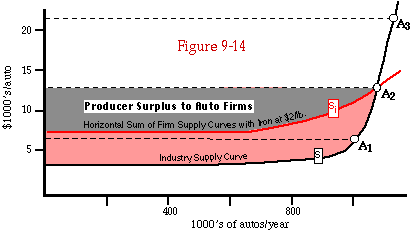Industry supply curves with (S) and without (Si) effects on input prices. The producer surplus calculated from Si is equal to the summed producer surpluses of the ten firms of Figure 9-13a. It is less than the producer surplus calculated from S; the difference represents producer surplus going to the iron industry of Figure 9-13b.

By introducing the possibility that the industry may have to pay a higher price for its inputs if it consumes more of them, I have considerably complicated the problem; I could have saved both myself and you a good deal of work by assuming the problem away, just as I assumed, for the purposes of this chapter, that variations in output by a single firm did not affect the prices at which it sold and bought. The reason I did not do so is that, in exchange for the additional complications of Figure 9-13a, we get two important results. One will be postponed to the next section; the other will be discussed here.

Back in Chapter 5, we saw that the producer surplus calculated from a supply curve representing the total supply of several producers was the same as the sum of the producer surpluses for all the individual supply curves of the individual producers. This is true for Figure 9-12; the industry supply curve is simply the firm supply curve multiplied horizontally by 10, so the producer surplus (profit) of the industry at any price is ten times the surplus of the firm. It does not, however, appear to be true for Figure 9-13a.

Suppose the price of autos is P2. The auto industry produces a quantity Q2 (point A2 on Figure 9-13a). It consumes a quantity QI2 of iron at a price of \$2/lb. At this price the firm's supply curve is S2, which is identical to Sf on Figure 9-12 and so implies the same amount of producer surplus. But the industry supply curve S on Figure 9-13a is not the same as Si on Figure 9-12, as you can see on Figure 9-14, which shows both. S and Si intersect at point A2 where price is P2 and quantity is Q2. Since S is steeper than Si, the corresponding producer surplus at a price of P2 (the colored and gray regions on Figure 9-14) is larger than the producer surplus at the same price calculated from Si (the gray region). If the producer surplus for Si is ten times that for Sf, the producer surplus for S must be more than ten times that for S2. But S2 is the supply curve faced by a firm in the situation described by point A2 (price of iron = \$2/pound). There are ten such firms. It appears that the producer surplus of the industry is greater than the producer surplus of the firms that make it up! What have we missed?

The answer is on Figure 9-13b. The firms shown on Figure 9-13a are not the only producers who benefit from their output--there are also the producers of iron. The higher the quantity produced on Figure 9-13a, the higher the quantity of iron used--and the price (on Figure 9-13b) at which it sells. If we had drawn the figure precisely and to scale, using actual production functions, the colored area on Figure 9-13b, representing the producer surplus received by the producers of iron when the price of iron is \$2/pound, would just make up the discrepancy between the total producer surplus calculated from S on Figure 9-13a and the producer surplus per firm calculated from S2.

I have asserted this result: I have not proved it, nor will I in this book. Figures 9-12 through 9-14 and the discussion of the last few paragraphs should make the result seem plausible, since they demonstrate that the discrepancy exists and that it is the result of the same fact--a rising supply curve for iron--which is responsible for the existence of producer surplus on Figure 9-13b. But a plausibility argument is not a proof.

Free Entry and the Industry Supply Curve

So far, I have considered an industry with a fixed number of firms; in that context, the supply curve of the industry is simply the horizontal sum of the supply curves of the individual firms, with appropriate allowance for the way in which the firm supply curves shift if changes in the industry's output affect the price of its inputs. It is now time to drop the assumption that the number of firms in the industry is fixed and consider an ordinary competitive industry with free entry; anyone who wishes may start a firm.

Now, when price increases, not all of the resulting increase in output need come from existing firms; some may come from new firms started to take advantage of the higher price. Hence the industry supply curve, which tells us how total output responds to changes in price, is not simply the firm supply curve multiplied by the number of firms. This is the same situation we encountered in Chapter 5, when we noted that as the price of a good increases, more and more people find that they are better off producing it than producing anything else, so a higher price results in output from new producers as well as increased output by those already producing that good. Seen from the standpoint of this chapter, the new producers of Chapter 5 are new one-person firms entering the industry.

The simplest way to derive an industry supply curve is to assume, as in the previous section, that existing firms all have the same production function and that there exist an unlimited number of potential firms each with the same production function as the existing firms. Just as at the beginning of the previous section, we will start by ignoring any effect that the actions of the industry may have on the price of its inputs.

In that situation, the industry supply curve is very simple. If existing firms are making positive profits--if their total revenue is larger than their total cost--it will pay new firms to come into existence. As new firms come into existence, supply expands, driving down the price. The process continues until profit is no longer positive. If, on the other hand, existing firms are making negative profits, then firms go out of business, reducing supply and driving price up--until profit is no longer negative. The equilibrium point is where profit is zero.

There is only one possible equilibrium price--the price at which revenue exactly covers cost. If revenue exactly covers cost, then average cost must be equal to price. We know, from our analysis of the supply curve of the firm, that each firm is producing an output for which marginal cost equals price. So the equilibrium of the whole industry occurs where price, marginal cost, and average cost are all equal.

If marginal cost equals average cost, then, as we saw earlier in the chapter, average cost is at a minimum (or a maximum, a possibility we shall for the moment ignore). Hence the equilibrium of the industry has each firm producing at minimum average cost and selling its product for a price that just covers all costs. That implies that the supply curve for the industry is a horizontal line at price equal to minimum average cost, as shown in Figure 9-15a. Increases in demand increase the number of firms and the quantity of output, with price unaffected. We have described a constant-cost industry--one for which the cost of an additional unit of production is independent of quantity.

You may be puzzled by the assertion that new firms come into existence as soon as existing firms start making a profit; surely entrepreneurs require not merely some profit but enough to reimburse them for the time and trouble of starting a new firm. But profit is defined, by economists if not by accountants, as revenue minus cost, where cost includes the cost to the entrepreneur of his own time and trouble. Hence if firms are making greater than zero profits, they are more than repaying their owners for the costs of starting them.

There is another way in which the ambiguity in the term profit"can lead to confusion here; it is most easily illustrated in the case of a company owned by its stockholders. For accounting purposes, the profit of such a firm is what is left after paying for labor, raw materials, and the interest on money borrowed by the firm; it is what the stockholders get in exchange for their investment. For economic purposes, however, the capital provided by the stockholders must also be considered an input, and its opportunity cost--what the stockholders could have gotten by investing the same money elsewhere--is one of the costs of production. The firm makes an economic profit only if its profit in the accounting sense is enough to more than just pay the stockholders for the use of their capital--to give them a return greater than the normal market return on the amount they invested in the firm. Such a firm is more attractive than alternative investments. So if firms in an industry are making positive economic profit, new firms enter that industry, driving the price down to the point where economic profit is again zero.

Two Roads to an Upward-Sloped Supply Curve

The supply curves that I described in Chapters 5 and 7 sloped up; the higher the price, the higher the output. The analysis of this chapter seems to imply a horizontal supply curve, with unlimited output available at one price, as shown in Figure 9-15a. What have we left out?

In discussing the supply curve of an industry with free entry, we have ignored the effect of increases in the size of the industry on the price of its inputs. It is now time to stop doing so. If the output of automobiles increases, so does the demand for steel, auto workers, and Detroit real estate. As the demand for these things increases, their prices rise. As the price of the inputs increases, so does average cost; the result is a rising supply curve. Figure 9-15b shows this; it corresponds to Figure 9-13a of the previous section--for an increasing-cost industry instead of a constant-cost industry.Deducing an industry's supply curve from a firm's supply curve in an industry with open entry. Figure 9-15a shows the case in which the industry's inputs are in perfectly elastic supply; Figure 9-15b shows the case where they are not.

What are the differences between the two situations--a competitive industry with open entry and a competitive industry with closed entry? One, which can be seen by comparing Figure 9-13a to Figure 9-15b, is in the relation between the firm supply curve and the industry supply curve. In Figure 9-15b, the individual firm is always at the bottom of its supply curve--receiving a price equal to average cost and making no economic profit. Increased price causes increased quantity not by sliding the firms up their supply curves but by pulling new firms into the market.

The other difference can be seen if we also look at Figure 9-15a. In the previous section, where we considered an industry with a fixed number of firms, the supply curve sloped up even before we took account of the effect of the industry on the prices of its inputs. In this section, it does not. In that section, the effect of a rising supply curve for the industry's inputs was to make a rising supply curve for its outputs rise more steeply than it otherwise would; in this section, it is to make a flat supply curve for the industry's outputs into a rising one.

In comparing the two sections, it is also worth noting the relevance of the earlier discussion of producer surplus to the situation discussed here. In a competitive industry with free entry, profit is competed down to zero, so the firms receive no producer surplus. But if the industry supply curve slopes up, the industry as a whole must have producer surplus--shown, for a price of P2, as the shaded area on Figure 9-15b. The explanation is that all of the producer surplus passes through the firms to the suppliers of their inputs. If the suppliers are themselves competitive firms with free entry, it passes through them to their suppliers, until it eventually ends up in the hands of the ultimate suppliers--workers renting out their labor, landowners renting out their land, and so forth. This is a point that will become important in Chapter 14, where I discuss how incomes are determined by ownership of the factors of production--the ultimate inputs.

So far, I have explained upward-sloping supply curves, in the context of a competitive industry with free entry, as a result of upward-sloping supply curves for the industry's inputs. An alternative approach is to assume that some firms have access to "better" means of production than others, giving them better production functions. As the price rises, worse and worse firms are pulled into the market, with higher and higher minimum average costs. The price, at any level of production, must be high enough to cover the costs of the highest cost firm that is producing--the marginal firm--otherwise it will not produce. It must not be high enough to cover the costs of the next higher cost firm, the most efficient firm that is not producing--otherwise that firm would enter the market too. At a price at which marginal firms cover their costs, firms with lower costs than the marginal firms make net profits, unlike the zero-profit firms of the earlier analysis. Figure 9-16 shows how such a situation can be graphed. At a price P, firm 1, with the lowest cost curves, makes positive profits, shown by the colored area; firm 2 just covers its costs, and firm 3 has not yet come into existence.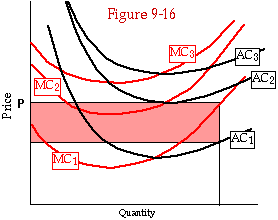An industry in which different firms have different cost curves. Firm 1, with average cost AC1 and marginal cost MC1, is making positive profits. Firm 2 is the marginal firm and makes zero profit. Firm 3 does not exist; it is a potential firm that would come into existence only at a higher price.

These two ways of getting upward-sloping supply curves are really the same. The reason that input costs eventually rise with increasing demand for inputs is that there is not an unlimited supply of identical inputs. There are only so many skilled widget makers willing to work for \$8/hour. To get more, you must pay more, inducing those presently employed to work more hours and luring additional workers into the industry. The same applies to land, raw materials, and capital goods. The reason firms do not all have the same cost curves is that some possess inputs that others lack--a particularly skilled manager, an unusually good machine, a favorable location. It is the limited supply of those particular inputs which implies that increased production must use worse machines, less skillful managers, worse locations--or pay more in order to attract high-quality inputs away from wherever they are presently being used.

So long as the scarce inputs actually belong to the firm--consisting, for instance, of the talents of the firm's owner or real estate belonging to a corporation--the distinction between having a better production function and having scarce assets may not be very important. Seen one way, the firm receives positive profits from its operations and turns them over to its owners; seen the other, its profits are zero, but its owners receive income on scarce resources that they rent to the firm. It is a more important distinction when the scarce asset belongs to the firm's landlord or one of its employees; when the relevant contracts are next renegotiated, the firm is likely to find that its positive profit was purely a short-run phenomenon.

Summing It Up

We have spent most of this chapter deriving the supply curve for an industry made up of many firms; the process has been sufficiently lengthy and contained enough complications and detours that you may well have lost track of just how we did it. This is a convenient place to recapitulate.

We start with a production function--a description of what quantity of output could be produced with any bundle of inputs. For any given set of input prices, we then calculate a total cost curve by finding the cost of the least expensive bundle of inputs necessary to produce each level of output. From that total cost curve--total cost of production as a function of quantity produced--we calculate average cost and marginal cost curves. From those we calculate a supply curve for the firm; each firm maximizes its profit by producing that quantity for which marginal cost equals price--unless, at that quantity, price is still below average cost, in which case the firm produces nothing and exits the industry.

Once we have the supply curve for the firm, we are ready to find the supply curve for the industry. If the industry is closed--new firms are not permitted--the supply curve for the industry is simply the horizontal sum of the supply curves of the firms that make it up, with some possible complications due to the effect of the quantity that the industry produces on the price of its inputs. If the industry is open--new firms are free to enter--then in equilibrium, profit must be zero, since positive profit attracts firms into the industry, driving down the market price, while negative profit drives firms out, raising the market price. In the simplest case--an unlimited supply of identical firms with horizontal (perfectly elastic) supply curves for their inputs--the result is a horizontal supply curve for the industry's output at a price equal to the minimum average cost of the firm. In the more complicated cases, the result is a rising supply curve. Price is still equal to minimum average cost--or if firms are not identical, it is between the minimum average cost of the highest cost firm that is producing and the minimum average cost of the lowest cost firm that is not.

Industry Equilibrium and Benevolent Dictation

The industry equilibrium we have just described--competitive equilibrium with free entry--has some interesting features. Suppose you were appointed dictator over the industry and told to produce the same output at the lowest possible cost. You would arrange things just as they are arranged in this solution--with each firm producing at minimum average cost.

From your standpoint, controlling the whole industry, there are two marginal costs for increasing output, corresponding to two different margins on which output can increase. One is the margin of the number of firms--output can be increased by having more firms. The cost of the extra units you get by adding an additional firm to the industry is that firm's average cost, so that is the marginal cost to the industry of increasing output on that margin. The other way of increasing output is by having each firm produce one more unit; the cost of those extra units is the firm's marginal cost. Marginal cost is the same on both margins--and must be if goods are being produced at minimum total cost. This is precisely analogous to the argument that showed that the marginal utility per dollar produced by different goods being consumed must be the same if utility is being maximized--it is one more application of the equimarginal principle. I leave the proof as an exercise for you; it is essentially the same as the last two or three times.

Another interesting feature of the competitive equilibrium is that price equals marginal cost; this implies that the price of a widget to a consumer deciding whether to consume one more is equal to the cost of producing it. He will choose to consume it only if it is worth at least that much to him--in which case it is, in some sense, "worth producing." This point will be discussed more precisely and in much more detail in Chapters 15 and 16.

Production and Exploitation

There is a sense in which nothing is produced. The laws of physics tell us that the sum total of mass and energy can be neither increased nor reduced. What we call "production" is the rearrangement of matter and energy from less useful to more useful (to us) forms.

It is sometimes said that only factories are really productive; middlemen (retailers and wholesalers) merely "move things about" while absorbing some of what others have produced. But all anyone does is to move things about--to rearrange from less to more useful. The producer rearranges iron ore and other inputs into automobiles; the retailer rearranges automobiles on a lot into automobiles paired up with particular customers. Both increase the value of what they work on and collect their income out of that increase.

It is often said that some participants in the economy "exploit" others--most commonly that employers exploit workers. This raises the question of what it means to exploit someone. Two different definitions are often used--simultaneously--in such discussions. The first is that I exploit you if I benefit by your existence. In this sense, I hope to exploit my wife and she hopes to exploit me; so far we have both succeeded. If that is what exploitation means, then it is the reason that humans are social animals and not, like cats, solitary ones.

The second definition is that I exploit you if I gain and you lose by our association. The connection between the two can be made either by claiming that the world is a "zero-sum game" in which the only way one person can gain is at another person's expense, or by arguing that if I gain by our association you deserve to have the gain given to you, so my refusal to give it to you injures you. The former argument is implausible. The second has a curious asymmetry to it. If I give you all the gain, you have now gained by our association and should obviously give it all back to me. It may be more sensible to keep the term exploitation out of economics and reserve it for political invective.

#### THE PUZZLE OF THE FIRM

Our analysis so far has shown how individuals coordinate their actions through the price system. This raises the question of why any other method is used. Why do firms exist? Why do we not observe an economy in which all producers are individuals, contracting with each other to buy and sell specific goods and services. Why do we observe instead firms, which buy people's time and then tell them what to do with it? Why is the capitalist beach made up of socialist grains of sand?

The simplest answer is that contracting can be costly. In Chapter 6, I described how bilateral monopoly (one buyer, one seller) can lead to costly bargaining as each party tries to get for himself as much as possible of the difference between the value of the good to the seller and to the buyer. While bilateral monopoly is in one sense rare, it is in another sense ubiquitous.

Consider a professor looking for a new job. There are, we will suppose, 20 universities as well suited to me as UCLA, and 200 economists as suitable for UCLA to employ as I am. Suppose I accept a job at UCLA, move to Southern California, buy a house, and spend a year or two learning to know and work with my colleagues and discovering how to teach UCLA undergraduates (by slipping lecture cassettes into their Sony Walkmen). When I came to UCLA, my salary was (say) \$30,000/year. Two years later, I am just as productive as expected and enjoy UCLA exactly as much as I expected to. But a problem arises.

The chairman of the department realizes that if I was willing to come for \$30,000, even though I had to pay the costs of moving and adjusting, then I would probably stay even if he reduced my salary to \$25,000--after all, there is no way I can get my moving expenses back by leaving. He calls me into his office to discuss the tight state of the department's budget.

I am glad to have a chance to talk to the chairman, for I too have been considering the situation. For my first two years, my productivity was reduced by the need to learn the ropes at my new job. If they were willing to offer me \$30,000/year, it was probably because, although I was really worth only \$25,000/year for the first two years, they expected me to be worth enough more than \$30,000/year thereafter to make up for the initial loss. Now that I have an opportunity to talk to the chairman, I will explain that, after considering the difficulty of the work I am doing, I believe I am entitled to a substantial raise. After all, there is no way he can get back the money he has lost on me during the first two years.

What we have here is a situation that was initially competitive but became a bilateral monopoly (with potential bargaining costs) once the trading parties had made costly adjustments to each other. The obvious solution is long-term contracting. When I come to UCLA, it is with an agreement specifying my salary for some years into the future.

This solution is itself costly--it constrains us even if circumstances change so that the contract should be renegotiated. There is no easy way to distinguish renegotiation motivated by a change in circumstances from renegotiation designed to take advantage of the bilateral monopoly created by our adjustments to each other. We could try to make the salary contingent on relevant circumstances (cost of living, university budget, alternative job offers), but there will never be enough small print to cover all of them.

The firm is a particular sort of long-term contract, in which the workers agree to do what they are told (within certain limits) for a stated number of hours a day in exchange for a fixed payment. The central problem of the firm is summed up in the Latin phrase qui custodes ipsos custodiet--"Who guards the guardians?" Since the workers receive a fixed wage, their objective is to earn it in the most enjoyable way possible; this is not necessarily the same behavior that maximizes the firm's profits. It is necessary to hire supervisors to watch the workers and make sure they do their job. Who then is to watch the supervisors? Who is to watch him?

One answer is to have the top supervisor be the residual claimant--the person who receives the firm's net revenue as his income. He watches the supervisors below him, they watch the ones below them, and so on. The residual claimant does not have to be watched in order to make him act in the interest of the firm--his interest and the firm's interest are the same.

What I have described is a firm run by its owner. This is a common arrangement in our economy. It makes sense in a situation where the worker whom it is most difficult for anyone else to supervise is the top supervisor; since he is the residual claimant, he supervises himself. While it is a common arrangement, it is not a universal one; not all firms are managed by their owners. In some, the worker whom it is most difficult and important to supervise is not the top manager but some skilled worker on whose output the firm depends--an inventor, for instance, with a firm built around him to support his genius (Browning, Ruger, Dolby). It may make sense for him to be the residual claimant--the owner of the firm--and for the top manager to be an employee; that is how such firms are sometimes organized. In other firms, there may be a group of skilled workers who can most easily be supervised by each other. You then get a workers' cooperative, although not necessarily one that includes all of the workers. An example is a law partnership.

There is another common solution to the problem of organizing a firm--a joint stock corporation, owned neither by its managers nor by its workers but by the stockholders who provide much of its capital, and controlled by the managers that those stockholders elect. Considering that solution brings us to some interesting problems--and a historical digression.

#### Even Homer Nods: Smith and the Corporation

Adam Smith, who in the eighteenth century produced the most influential economics book ever written, argued that corporations were almost hopelessly incompetent. With ownership widely dispersed, everybody's business is nobody's business; the managers can do what they like with the stockholders' money. Smith predicted that corporations would succeed only with government support, except in areas that required large amounts of money and very little skill--such as banking and insurance.

Smith was wrong; even where they have no special support from government (save for the privilege of limited liability)--even when government imposes special taxes on them--corporations have successfully competed with owner-run firms and partnerships in a wide range of fields. His mistake was in failing to predict the benign effects of the take-over bid.

Imagine you know that a corporation is being badly run. You buy as much stock as possible--enough to let you take over the corporation and install competent managers. Earnings shoot up. The market value of your stock shoots up. You sell out and look for another badly managed firm. Such behavior is discouraged by securities regulation and vituperated by existing managements, for obvious reasons. It (and its threat, which helps keep managers honest) may be the reason for the success of the corporation in the modern world.

This raises an interesting idea. The same arguments that show that the corporation cannot work apply with still greater force to democratic government. In a presidential election, the individual voter has one chance in several million of deciding the outcome--so why should he spend valuable time and energy studying the candidates and the issues before he votes? Here again, everybody's business is nobody's business. The result is that most voters do not even know the names of many of the politicians who "represent" them.

Is there a reason why the solution to the problems of the corporation--the take-over bid--does not solve the problems of democratic government? Yes. The difference between the two cases is that your "share" in the United States is not transferable property--which may be why, if casual observation is to be trusted, democratic governments are worse run than most corporations.

Your share in the United States is not transferable property--but perhaps it could be. Imagine that it were. Each citizen owns one citizenship, which includes one vote. You may leave the country and sell your citizenship to someone who wants to live here. If the country is badly run, someone can buy up a vast number of citizenships, elect a competent government, and make a fortune reselling the citizenships at a higher price. The country need not be emptied while the operation is going on; the operator can always rent his citizenships out between the time he buys them and the time he sells them.

#### PRODUCTION FUNCTION TO COST CURVE VIA CALCULUS

At the beginning of this chapter, I described the production function of a firm producing clay pots and showed how it could be used to find the total cost curve. One problem with the procedure described there was that Table 9-1 showed only a few of the possible bundles of inputs. Looking over the alternative bundles shown on the table to find the least costly way of producing any level of output only guarantees that you end up with the best alternative among those shown; there may be other bundles, not shown on the table, that are even less costly. Another problem is that the table shows bundles for producing only a few of the many possible levels of output.

Both problems can be eliminated if we use calculus instead of trial and error. The production function, which is given at the bottom of Table 9-1, tells us how much output we can produce from any combination of inputs:

Q = L1/2 (K/100)1/4R1/4. (Equation 1)

Here Q is the quantity of output (number of pots), L the amount of labor, K the amount of capital, and R the amount of raw material (clay). Since, according to Table 9-1, the price of labor is \$10/hour, the price of capital is .05/year (an interest rate of 5 percent), and the price of clay is \$4/pound, the cost (C) of any bundle of labor, capital, and raw material is:

C = 10L + .05K + 4R. (Equation 2)

Our problem is to find the values of L, K, and R that minimize C for a given Q.

The first step is to use Equation 1 to eliminate one of the variables. Rearranging the equation, we have:

R = 100Q4/L2K. (Equation 3)

Substituting that into Equation 2 gives us:

C = 10L + .05K + 400Q4/L2K. (Equation 4)

Minimizing Equation 4 by varying K and L while holding Q constant gives us two first-order equations:

0 =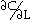= 10 - 800Q4/L3K

and

0 =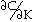= .05 - 400Q4/L2K2.

Solving those, we have:

L3K = 400Q4/5 = 80Q4 (Equation 5)

and

L2K2 = 40,000Q4/5 = 8,000Q4. (Equation 6)

Taking the square root of Equation 6 gives us:

LK = 200Q2/51/2. (Equation 7)

Dividing Equation 5 by Equation 7 gives us:

L2 = 2Q2/51/2.

Solving for L, we have:

L = 21/2Q/51/4=.946 Q.

We can then plug that into Equation 7 and solve for K:

K = 20Q x 21/253/4= 94.6 Q.

We then find R by plugging K and L into Equation 3; the result is:

R = Q(53/4/23/2)= 1.182 Q.

We now have L, K, and R as functions of Q. For any value of Q, they tell us how much of each input is included in the least-cost bundle that can be used to produce that quantity of output; mathematical purists may wish to check the second-order conditions to make sure we have minimized cost instead of maximizing it. Inserting the expressions for L, K, and R into Equation 2 gives us the total cost curve--the cost of producing any quantity of output in the least expensive possible way.

TC(Q) = 10(.946Q) + .05(94.6Q) + 4(1.182Q) = Q(9.46+4.73+4.73)

= 18.92 Q

Bundles O, P, Q, and R on Table 9-1 show the results of solving for Q = 1, 2, 3, and 4; Figure 9-1 shows the total cost curve.

#### Production Functions and Returns to Scale

In analyzing the production of pots, our production function was:

Q(L,K,R)=L1/2 (K/100)1/4R1/4

This is an example of a type of production function, called a Cobb-Douglas production function (after economist Paul Douglas and mathematician Charles Cobb), that is frequently used in economic theory--not because it describes actual firms better than alternative functions but because it has some convenient mathematical properties. The general Cobb-Douglas production function, for inputs X, Y, Z, ... is:

Q(X, Y, Z, ...) = AXaYbZc... (Equation 8)

Consider a function of this form for which the sum of the exponents equals 1; for simplicity I assume only three inputs:

a+b+c=1 (Equation 9)

We have:

Q(kX, kY, kZ) = A(kX)a(kY)b(kZ)c=Ak(a+b+c)XaYbZc=kQ(X, Y, Z)

Put in words, this tells us that if the exponents sum to 1, then multiplying all inputs by a constant multiplies the output by the same constant. If we use twice as much labor, and steel, and rubber, we produce twice as many automobiles. A further result, which you could check by redoing our analysis of the pottery production function (Equation 1) for the more general case shown in Equations 8 and 9, is that as the amount you want to produce increases, the amount of each input in the optimal production bundle increases proportionally. If using particular quantities of labor, steel, and rubber is the lowest cost way of producing 100 automobiles, then using ten times those quantities is the lowest cost way of producing 1000.

Such a production function exhibits constant returns to scale. The corresponding total cost curve is a straight line through the origin; the average and marginal cost curves are horizontal and identical. Average cost is the same however many units you make.

If the exponents of a Cobb-Douglas production function sum to less than 1, doubling all inputs results in less than a doubling of output (decreasing returns to scale). If the exponents sum to more than 1, doubling inputs more than doubles outputs (increasing returns to scale). In all of these cases, just as in the constant returns to scale case, the ratio of the different inputs in the optimal input bundle stays the same as the scale of output increases. If 10 units of X, 20 units of Y, and 15 units of Z make up the least cost bundle for producing 100 widgets, then 20 units of X, 40 units of Y, and 30 units of Z is also a least cost bundle. Whether that bundle produces 200 widgets (constant return to scale), fewer than 200 (decreasing returns to scale) or more than 200 (increasing returns to scale) depends on the sum of the exponents in the production function.

This implies that, for a Cobb-Douglas production function, decreasing returns to scale (if all inputs are increased by the same factor, output increases by a smaller factor) imply net diseconomies of scale (the cost of producing a given quantity of output, using the least cost bundle, increases with quantity) and increasing returns to scale imply net economies of scale. For other production functions that relationship might not be true. One could imagine a case where doubling all inputs resulted in a less than doubling of output, but where doubling expenditure on inputs (and changing the mix of inputs) resulted in a more than doubling of output. Consider, for instance, a situation where one of the costs of production is designing the product; it is not necessary to double the input of designers in order to double the number of units of output produced.

#### PROBLEMS

1. My production function for grading finals is:

F=L1/2O1/2

where F is the number of finals graded, L is my labor, and O the number of hours I must spend at my optometrist's to make up for the damage done to my eyes. My labor grading exams is worth \$15/hour (writing textbooks is more fun), and the optometrist charges \$45/hour.

a. Draw isoquants for F=10, F=40.

b. Draw isocost lines for expenditures of \$600 and \$1200.

c. Draw my total cost curve from F=10 to F=100. You may use trial and error (a spreadsheet helps), isoquants, or calculus, but you should find at least three points on the total cost curve.

2. Figure 9-17 shows isoquant curves for producing illuminated manuscripts; the inputs are parchment (price P=20)and labor (price W=1).

a. What is the least cost way of producing 10 manuscripts?

b. Show, as a table or a graph, how the number of parchments and hours used varies as the number of manuscripts produced goes from 5 to 32.

c. Manuscripts can be sold for \$40 apiece. About how many does the firm produce? How much labor does it hire? How many parchments does it buy?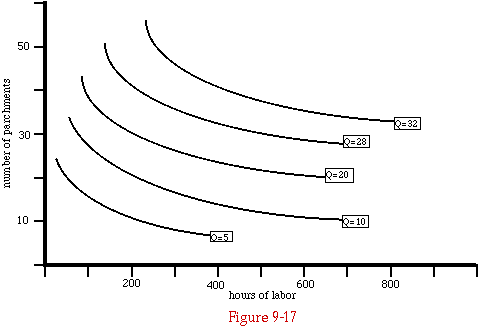Isoquant curves from a scriptorium, for Problem 2.

3. Figure 9-18 shows the average and marginal cost curves for a firm. At a price of \$6/widget, about how many widgets will the firm produce?

4. If additional firms like this are free to enter the market, what will the price of widgets eventually be?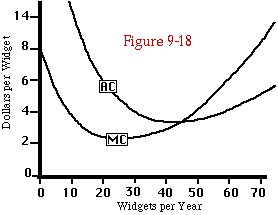Cost curves for Problems 3 and 4.

5. Figure 9-19a shows a total cost curve (total cost of producing widgets as a function of quantity of widgets produced). Which of the curves shown in Figure 9-19b could be the corresponding marginal cost curve? Which could be the corresponding average cost curve? (The vertical axes of the figures are deliberately left unmarked; answering the question does not require that information.)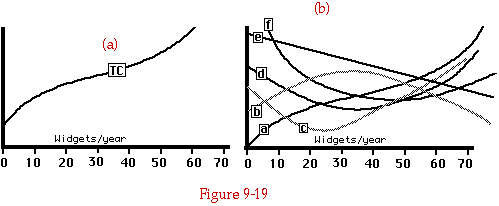Cost curves for Problem 5.

6. Figure 9-20 shows several pairs of MC and AC curves. Which pairs are possible? Which curve in the possible pairs is which? Explain.

7. How does the relation between MC and AC tell you whether AC is at a maximum or a minimum?

8. Demonstrate that the firm always prefers the point where MC intersects P from below to the point where it intersects it from above. What does this imply about the situation where marginal cost crosses average cost at the maximum instead of the minimum of average cost?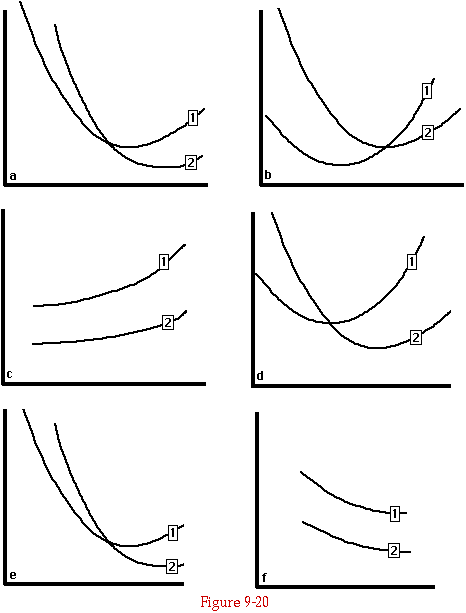Cost curves for Problem 6.

9. Figure 9-22 shows the supply curves for three types of potential firms--type 1, type 2, and type 3. Assume there are 10 of each type; no additional firms are allowed to enter the market. Draw the industry supply curve. Assume that all of the industry's inputs have horizontal supply curves; the amount purchased does not affect the price.

10. What are the essential differences between the analysis of production in this chapter and of consumption in Chapters 3 and 4?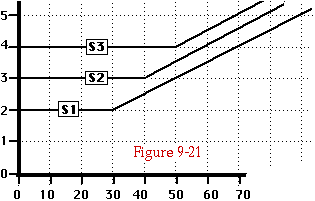Supply curves for Problem 9.

11. Figure 9-22 shows the supply curve for a pencil firm and the producer surplus that the firm receives if the price at which it can sell pencils is \$0.10/pencil. As in several earlier figures, the supply curve is discontinuous; there is no price at which the firm chooses to produce more than zero and fewer than 10,000 pencils per week. The supply curve shows, for any price, the quantity the firm chooses to produce at that price, so in the range of quantity between 0 and 10,000, the supply curve does not exist.

Producer surplus is the area above the supply curve and below the price. In this case, between 0 and 10,000 pencils per week, there is no supply curve for it to be above. Nonetheless, here and earlier, the region representing producer surplus is drawn as if the supply curve had a horizontal section at the discontinuity--as if, in other words, the dashed line on the figure were really part of the supply curve.

Prove that this is the correct way of calculating producer surplus in this case. (This is a hard question.)

(Hint: You will want to use both the marginal cost and the average cost curves of the firm in your proof. Second Hint: What is producer surplus at a price of \$0.06/pencil? Why?)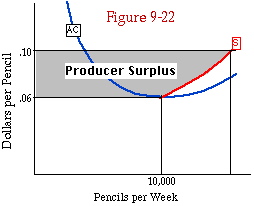Supply curve and producer surplus for Problem 11.

12. The friends who rent our third floor are enthusiastic gardeners; we are not. The result is that we get free gardening and they get free use of a yard to garden in. Who is exploiting whom, in which sense? What might be a better term to describe the situation?

13.Who or what do cats exploit? In which sense or senses of the word?

The following problems refer to the optional section.

14. The production function is the same as for Table 9-1; the price of labor is \$5/hour, the price of capital is .04/year, and the price of clay is \$6/pound. Find and graph the total cost curve.

15. Prices are the same as in Problem 14; the production function is:

Q = L1/3K1/3R1/3.

Solve for L, K, R, and TC as functions of Q, for 1<= Q<= 64.

16. Your production function is as in Problem 17. You have decided to produce 100 pots; you have already bought 8 pounds of clay, so the only question is how much labor and capital to use. The wage rate is \$10/hour, and the interest rate is 10 percent.

a. Use calculus to find the optimal values of L and K.

b. Solve the same problem using an isoquant-isocost diagram similar to Figure 9-2.